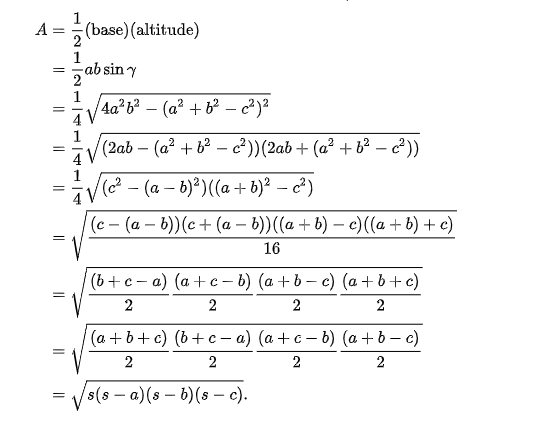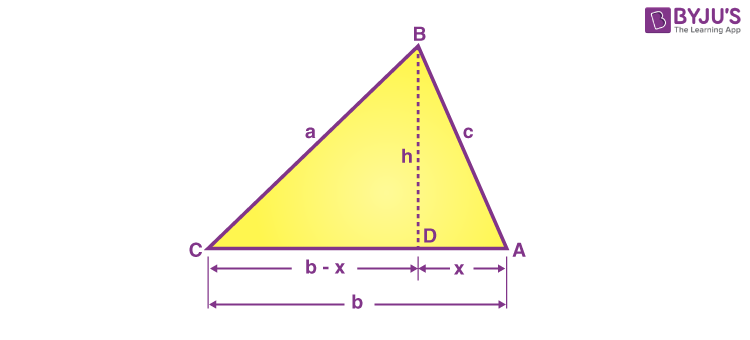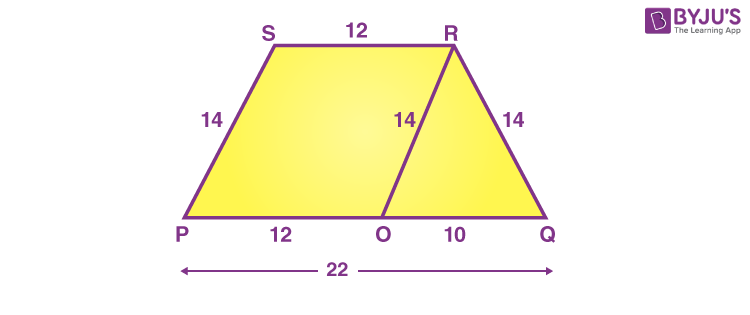# Heron's Formula

Heron’s formula is used to find the area of a triangle when we know the length of all its sides. It is also termed as Hero’s Formula. We can apply Heron’s formula to find different types of triangles, such as scalene, isosceles and equilateral triangles. We don’t have to need to know the angle measurement of a triangle to calculate its area, by using Heron’s formula.

## What is Heron’s Formula?

Heron’s formula is a formula to calculate the area of triangles, given the three sides of the triangle. This formula is also used to find the area of the quadrilateral, by dividing the quadrilateral into two triangles, along its diagonal.

If a, b and c are the three sides of a triangle, respectively, then Heron’s formula is given by:

 $$\begin{array}{l}Area~of~triangle~using~three~sides =\sqrt{s(s-a)(s-b)(s-c)}\end{array}$$   Semiperimeter, s = Perimeter of triangle/2 = (a+b+c)/2

## History of Heron’s Formula

Hero of Alexandria was a great mathematician who derived the formula for the calculation of the area of a triangle using the length of all three sides. He also extended this idea to find the area of quadrilateral and also higher-order polygons. This formula has its huge applications in trigonometry such as proving the law of cosines or the law of cotangents, etc.

## Heron’s Formula for Triangles

According to Heron, we can find the area of any given triangle, whether it is a scalene, isosceles or equilateral, by using the formula, provided the sides of the triangle.

Suppose, a triangle ABC, whose sides are a, b and c, respectively. Thus, the area of a triangle can be given by;

$$\begin{array}{l}Area =\sqrt{s(s-a)(s-b)(s-c)}\end{array}$$

Where “s” is semi-perimeter = (a+b+c) / 2

And a, b, c are the three sides of the triangle.

## Proof of Heron’s Formula

There are two methods by which we can derive Heron’s formula.

• First, by using trigonometric identities and cosine rule.
• Secondly, solving algebraic expressions using the Pythagoras theorem.

Let us see one by one both the proofs or derivation.

### Using Cosine Rule

Let us prove the result using the law of cosines:

Let a, b, c be the sides of the triangle and α, β, γ are opposite angles to the sides.

We know that,  the law of cosines is

$$\begin{array}{l} cos \gamma =\frac{a^2+b^2-c^2}{2ab}\end{array}$$

Again, using trig identity, we have

$$\begin{array}{l}sin \gamma = \sqrt{1-cos^2γ} \end{array}$$

$$\begin{array}{l}= \frac{\sqrt{4a^2b^2-(a^2+b^2-c^2)^2}}{2ab}\end{array}$$

Here, Base of triangle = a

Altitude = b sinγ

Now,### Using Pythagoras Theorem

Area of a Triangle with 3 Sides

Area of ∆ABC is given byA = 1/2 bh _ _ _ _ (i)

Draw a perpendicular BD on AC

x2 + h2 = c2

x2 = c2 − h2—(ii)

⇒ x = √(c2−h2)−−−−−−—(iii)

Consider a ∆CDB,

(b−x)2 + h2 = a2

(b−x)2 = a2 − h2

b2 − 2bx + x2 = a2–h2

Substituting the value of x and x2 from equation (ii) and (iii), we get

b2 – 2b√(c2−h2)+ c2−h2 = a2 − h2

b2 + c2 − a2 = 2b√(c2 − h2)

Squaring on both sides, we get;

(b2+c2–a2)2 = 4b2(c2−h2)

$$\begin{array}{l}\frac{(b^2 + c^2 -a^2 )^2}{4b^2} = c^2 – h^2\end{array}$$

$$\begin{array}{l}h^2~ = ~c^2 ~-~\frac{(b^2 +c^2 -a^2 )^2}{4b^2}\end{array}$$

$$\begin{array}{l}h^2~ =~\frac{4b^2 c^2 – (b^2 +c^2 -a^2 )^2 }{4b^2}\end{array}$$

$$\begin{array}{l}h^2~=~\frac{(2bc)^2 -(b^2+c^2- a^2 )^2}{4b^2}\end{array}$$

$$\begin{array}{l}h^2~=~\frac{[2bc+(b^2 +c^2 -a^2 )][2bc-(b^2+c^2-a^2)]} {4b^2}\end{array}$$

$$\begin{array}{l}h^2~ =~\frac{[(b^2+2bc+c^2)-a^2][a^2-(b^2- 2bc+c^2)]}{4b^2}\end{array}$$

$$\begin{array}{l}h^2~=~\frac{[(b+c)^2 – a^2 ].[a^2 -(b-c)^2 ]}{4b^2}\end{array}$$

$$\begin{array}{l}h^2~=~\frac{[(b+c)+a][(b+c)-a].[a+(b-c)][a-(b-c)]}{4b^2}\end{array}$$

$$\begin{array}{l}h^2~=~\frac{(a+b+c)(b+c-a)(a+c-b)(a+b-c)}{4b^2}\end{array}$$

The perimeter of a ∆ABC is given by:

P = a + b + c

Thus, substituting a + b + c = P in the above equation, we get;

$$\begin{array}{l}⇒~h^2~ =~\frac{P(P – 2a)(P – 2b)(P -2c)}{4b^2}\end{array}$$

$$\begin{array}{l}⇒~h~ = \frac{\sqrt{P(P – 2a)(P – 2b)(P -2c)}}{{2b}}\end{array}$$

Substituting the value of h in equation (i), we get;

$$\begin{array}{l}A~=~\frac{1}{2} b \frac{\sqrt{P(P – 2a)(P – 2b)(P -2c)}}{2b}\end{array}$$

$$\begin{array}{l}A~=~\frac{1}{4}\sqrt{(P(P – 2a)(P – 2b)(P -2c)}\end{array}$$

$$\begin{array}{l}A~=~\sqrt{\frac{1}{16} P(P – 2a)(P – 2b)(P -2c)}\end{array}$$

$$\begin{array}{l}A~=~\sqrt{\frac{P}{2}\left(\frac{P – 2a}{2}\right)\left(\frac{P – 2b}{2}\right)\left(\frac{P -2c}{2}\right)}\end{array}$$

$$\begin{array}{l}Semi\ perimeter(s) =\frac{perimeter}{2}~=~\frac{P}{2}\end{array}$$

$$\begin{array}{l}~A~=~\sqrt{s(s – a)(s – b)(s – c)}\end{array}$$

Note: Heron’s formula is applicable to all types of triangles and the formula can also be derived using the law of cosines and the law of Cotangents.

## How to Find the Area Using Heron’s Formula?

To find the area of a triangle using Heron’s formula, we have to follow two steps:

• Find the perimeter of the given triangle
• Then, find the value of the semi-perimeter of the given triangle; s = (a + b + c)/2
• Now use Heron’s formula to find the area of a triangle (√(s(s – a)(s – b)(s – c)))
• Finally, represent the area with the accurate square units (such as m2, cm2, in2, etc.)

### Heron’s Formula for Equilateral Triangle

As we know the equilateral triangle has all its sides equal. To find the area of the equilateral triangle let us first find the semi perimeter of the equilateral triangle will be:

s = (a + a + a)/2

s = 3a/2

where a is the length of the side.

Now, as per the heron’s formula, we know;

$$\begin{array}{l}Area =\sqrt{s(s-a)(s-b)(s-c)}\end{array}$$

Since, a = b = c

Therefore,

A = √[s(s – a)3]

which is the required formula.

### Heron’s Formula for Isosceles Triangle

An isosceles triangle has two of its sides equal and the angles corresponding to these sides are congruent. To find the area of an isosceles triangle, we can derive the heron’s formula as given below:

Let a be the length of the congruent sides and b be the length of the base.

Semi-perimeter (s) = (a + a + b)/2

s = (2a + b)/2

Using the heron’s formula of a triangle,

Area = √[s(s – a)(s – b)(s – c)]

By substituting the sides of an isosceles triangle,

Area = √[s(s – a)(s – a)(s – b)]

= √[s(s – a)2(s – b)]

Or

= (s – a)√[s(s – b)]

which is the required formula to find the area for the given isosceles triangle.

### Heron’s Formula for Scalene Triangle

As we know, a scalene triangle has all three unequal sides, thus, we can directly use the original Heron’s formula to find the area.

Area of scalene triangle = √s(s – a)(s – b)(s – c),

where s = (a + b + c)/2

Let us learn how to find the area of quadrilateral using Heron’s formula here.

If ABCD is a quadrilateral, where AB||CD and AC & BD are the diagonals.

Now we have two triangles here.

So, if we know the lengths of all sides of a quadrilateral and the length of diagonal AC, then we can use Heron’s formula to find the total area.

Hence, we will first find the area of ∆ADC and area of ∆ABC using Heron’s formula and at last, will add them to get the final value.

## Applications of Heron’s Formula

The two major applications of Heron’s formula are:

• To find the area of different types of a triangle (when the length of three sides are given)
• To find the area of a quadrilateral (when the length of all three sides are given)

## Solved Examples

Let us now look into some examples to have a brief insight into the topic:

Example 1: Find the area of a trapezium, the length of whose parallel sides is given as 22 cm and 12 cm and the length of other sides is 14 cm each.

Solution: Let PQRS be the given trapezium in which PQ = 22 cm, SR = 12 cm,

PS = QR = 14 cm.

Constructions: Draw OR||PS

Now, PORS is a parallelogram in which PS||OR and PO||SR

Therefore, PO = SR = 12 cm

⇒ OQ = PQ – PO = 22 – 12 = 10 cmIn ∆OQR , we have

$$\begin{array}{l}s~ = ~\frac{14+14+10}{2}~ =~\frac{38}{2}~=~19\end{array}$$

$$\begin{array}{l}\text {Area of ∆OQR} =\sqrt{s(s – a)(s – b)(s –C)}\end{array}$$

$$\begin{array}{l} =\sqrt{ (19(19 – 14)(19 – 14)(19 –10))}\end{array}$$

$$\begin{array}{l} = \sqrt{4275}\end{array}$$

$$\begin{array}{l}= 15 \sqrt{19} cm^2 ………(i)\end{array}$$

$$\begin{array}{l}\text{We know that Area} =\frac{1}{2} \times b \times h\end{array}$$

$$\begin{array}{l}\Rightarrow 15\sqrt{19} = \frac{1}{2} \times 10 \times h\end{array}$$

$$\begin{array}{l}\Rightarrow h = 5\sqrt{19} …………..(ii)\end{array}$$

$$\begin{array}{l}\text{Area of trapezium} = \frac{1}{2}~ (PQ+SR) × h \end{array}$$

$$\begin{array}{l}=\frac{1}{2}(22+12) × 3 \sqrt{19} \end{array}$$

$$\begin{array}{l}=51 \sqrt{19} cm^2\end{array}$$

Example 2: Find the area of the triangle whose sides measure 10 cm, 17 cm and 21 cm.  Also, determine the length of the altitude on the side which measures 17 cm.

Solution:

$$\begin{array}{l} s = \frac{a+b+c}{2} =\frac{10+17+21}{2}= 24\end{array}$$

$$\begin{array}{l}\text{Area of Triangle} =\sqrt{s(s-a)(s-b)(s-c)}\end{array}$$

$$\begin{array}{l}\sqrt{24 \times 14 \times 7 \times 3}\end{array}$$

$$\begin{array}{l}\sqrt{7056}= 84 \ square\ cm\end{array}$$

Taking 17 cm as the base length we need to find the height

Area, A = 1/2 × base × height

1/2  × 17 × h = 84   or h = 168/17 = 9.88 cm   (Rounded to the nearest hundredth).

Example 3: A triangle PQR has sides 4 cm, 13 cm and 15 cm. Find the area of the triangle.

Solution:

Semiperimeter of triangle PQR, s = (4 + 13 + 15)/2 = 32/2 = 16

By heron’s formula, we know;

A = √[s(s – a)(s – b)(s – c)]

Hence, A = √[16(16 – 4)(16 – 13)(16 – 15)] = √(16 × 12 × 3 × 1) = √576 = 24 sq.cm

### Heron’s Formula – Practice Questions

A few practice questions are given below that will help you in improving your knowledge of how to find the area of a given triangle.

1. Find the area of a triangle whose perimeter is 54 cm and two of its sides measure 12 cm and 25 cm. [Answer: 90 sq.cm]
2. If the length of equal sides of an isosceles triangle is 5 cm and base is 6 cm, then find its area using heron’s formula. [Answer: 12 sq.cm]
3. The sides of a quadrilateral field, taken in order are 26 cm, 27 cm, 7 cm, 24 cm respectively. The angle contained by the last two sides is a right angle. Find its area. [Answer: 375.85 sq.cm.]

### Related Articles

To solve more problems based on Heron’s Formula, Register with BYJU’S today and clear all your doubts on maths concepts.  We provide detailed and step-by-step solutions to all questions asked by you. Also, take free tests to practice for exams.

## Frequently Asked Questions – FAQs

Q1

### What does ‘s’ represent in Heron’s Formula?

The s in Heron’s formula denotes the semi-perimeter of a triangle, whose area has to be evaluated. Semi-perimeter is equal to the sum of all three sides of the triangle divided by 2.
s = (a + b + c)/2
Where a, b and c are three sides of a triangle.
Q2

### When do we use Heron’s formula?

Heron’s formula is used to find the area of a triangle when all its three side-lengths are known to us.
Q3

### Who gave Heron’s formula?

Hero of Alexandria was a great mathematician who derived the formula for the calculation of the area of a triangle using the length of all three sides.
Q4

### What is Heron’s formula for equilateral triangle?

Since an equilateral triangle has all its three sides equal, therefore, the hero’s formula to find its area is given by:
A = √[s(s – a)3]
Q5

### How can we find the area of quadrilateral using Heron’s formula?

If we know the lengths of the sides of a quadrilateral and any one of the diagonal length, then by taking diagonal as the common side, we can divide the given quadrilateral into two triangles and find the area for both using Heron’s formula. At last, we need to add the areas of the two triangles.
Test your knowledge on Heron Formula